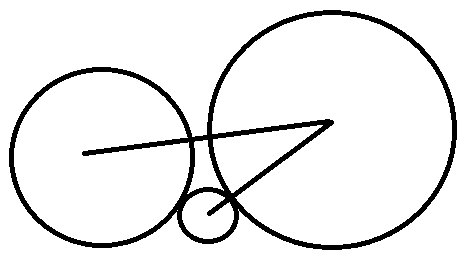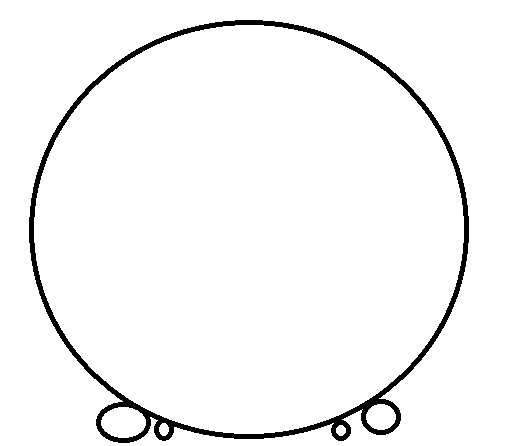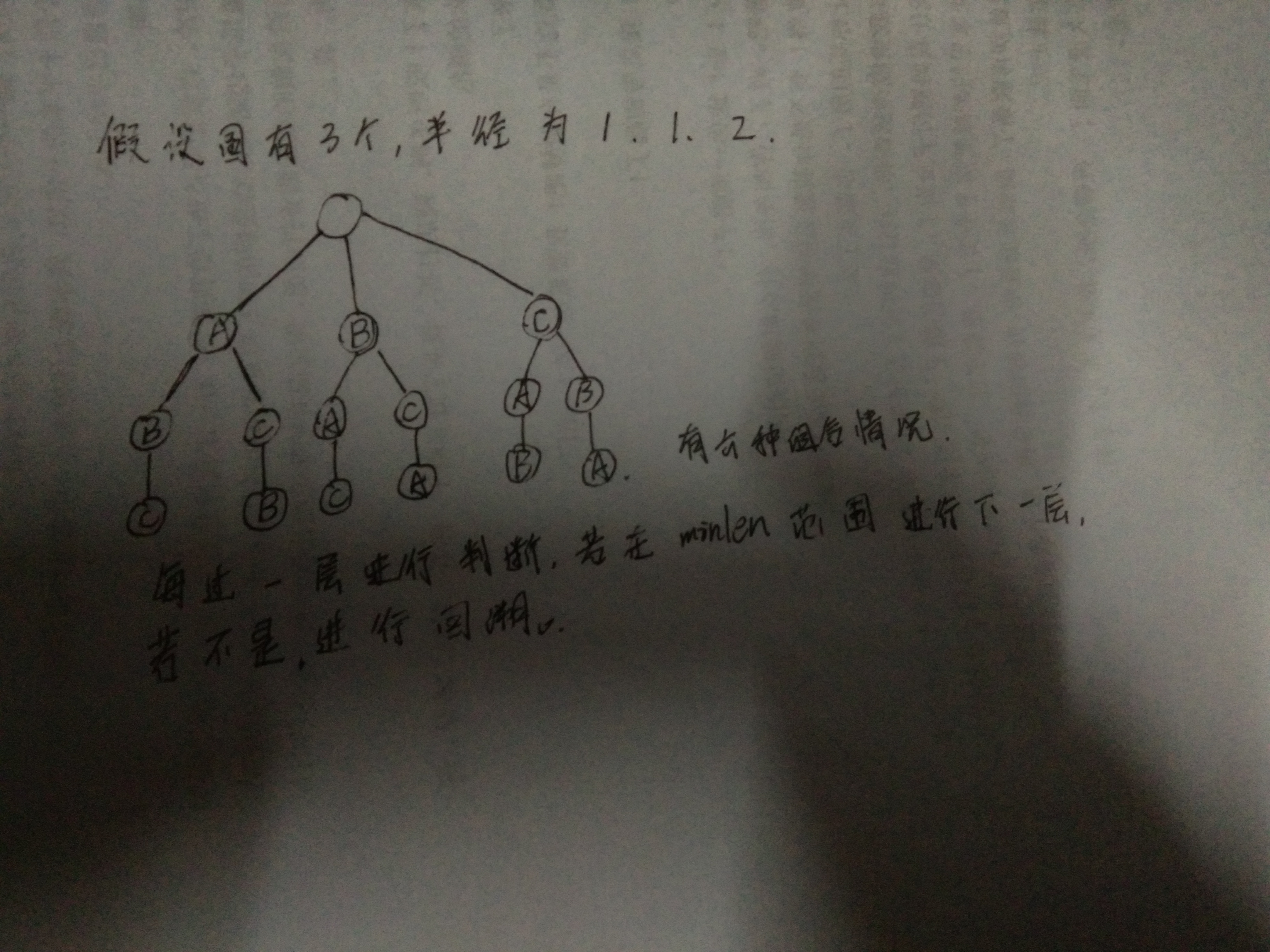## 圆排列问题_小张的java日记的博客-程序员宅基地_圆排列java

首先计算圆在当前圆排列中的横坐标，由x^2 = sqrt((r1+r2)^2-(r1-r2)^2)推导出x = 2 * sqrt(r1 * r2)。``````void compute(){//计算圆排列长度
double low=0,high=0;
for (int i = 1; i <= n; ++i) {//寻找最左端与最右端的距离
if (x[i] - r[i] < low) {
low = x[i] - r[i];
}
if (x[i] + r[i] > high) {
high = x[i] + r[i];
}
}
if (high - low < minlen) {
minlen = high - low;
for (int i = 1; i <= n; ++i) {
bestv[i] = r[i];
}
}
}
void Backtrack(int t){
if (t > n)
compute();
else{
for (int i = t; i <= n; ++i) {
//确保全排列：一开始按顺序的时候没交换，第一次排列后，回溯时i与t不同
swap(r[t], r[i]);
double centerx = center(t);//计算第t个圆的横坐标
if (centerx + r + r[t] < minlen) {//剪枝
x[t] = centerx;//确定了加入第t个圆的圆排列长度
Backtrack(t + 1);//搜索下一个圆
}
swap(r[t], r[i]);//回溯，将前面全排列结束后复原，再接着从更前一个元素开始排列
}
}
}
``````

``````#include<iostream>
#include<cmath>
#include<algorithm>
using namespace std;
const int MAXN = 100;
int n;//圆的个数
double minlen=1000000,x[MAXN],r[MAXN];//分别为最小圆排列长度，每个圆心横坐标数组，每个圆半径数组
double bestv[MAXN];//最小圆排列的半径顺序
double center(int t){//得到第t个圆的圆心横坐标
double tmp = 0;
for (int i = 1; i < t; ++i) {//计算第t个圆与前面(序号为1~t-1)已排列圆相切时的距离，求最大距离
double xvalue = x[i] + 2.0 * sqrt(r[i]*r[t]);//计算第t个圆与第i个圆相切时的距离
if (xvalue > tmp) {//最大的距离就是圆心坐标
tmp = xvalue;
}
}
return tmp;
}
void compute(){//计算圆排列长度
double low=0,high=0;
for (int i = 1; i <= n; ++i) {//寻找最左端与最右端的距离
if (x[i] - r[i] < low) {
low = x[i] - r[i];
}
if (x[i] + r[i] > high) {
high = x[i] + r[i];
}
}
if (high - low < minlen) {
minlen = high - low;
for (int i = 1; i <= n; ++i) {
bestv[i] = r[i];
}
}
}
void Backtrack(int t){
if (t > n)
compute();
else{
for (int i = t; i <= n; ++i) {
//确保全排列：一开始按顺序的时候没交换，第一次排列后，回溯时i与t不同
swap(r[t], r[i]);
double centerx = center(t);//计算第t个圆的横坐标
if (centerx + r + r[t] < minlen) {//剪枝
x[t] = centerx;//确定了加入第t个圆的圆排列长度
Backtrack(t + 1);//搜索下一个圆
}
swap(r[t], r[i]);//回溯，将前面全排列结束后复原，再接着从更前一个元素开始排列
}
}
}
int main()
{
cout << "圆的个数 n：";
cin >> n;
cout << "每个圆的半径分别为：";
for (int i = 1; i <= n; ++i) {
cin >> r[i];
}
Backtrack(1);
cout << "最小圆排列长度为：" << minlen <<endl;
cout << "最优圆排列的顺序对应的半径分别为：";
for (int i = 1; i <= n; ++i) {
cout << bestv[i] << " ";
}
return 0;
}
``````

### react-router4_虚年的博客-程序员宅基地

React-router41. 基础使用安装yarn add react-router-dom -S基本使用BrowserRouter 包裹整个应用Router路由对应渲染的组件，可嵌套Link跳转专用import { BrowserRouter, Route, Link } from 'react-router-dom'ReactDOM.render( <BrowserRouter>

### 【Hue】大数据WEB工具Hue_魏晓蕾的博客-程序员宅基地_hue是什么工具

Hue的安装及结合HDFS、Hive、RDBMS、Oozie的配置运行。

### hive 计算两个时间相差的分钟_轻风细雨的博客-程序员宅基地_hive计算两个时间差

select unix_timestamp(checktime,'yyyy-MM-dd HH:mm')- unix_timestamp(applytime,'yyyy-MM-dd HH:mm')/60,checkuser from tableau.home_person_check_time limit 10;

### java 6 update 3_Java SE 6 Update 23 正式发布_weixin_39652154的博客-程序员宅基地

Java SE 6 Update 23The full internal version number for this update release is 1.6.0_23-b05 (where "b" means "build"). The external version number is 6u23.HighlightsJava SE 6u23 contains enhancements ...

### ae渲染出现错误是什么问题_ae渲染提示渲染错误，渲染出现偏移解决方案_Lucy-Fintech社区的博客-程序员宅基地

AE输出序列时，提示渲染出现偏移，编译影片时出错，渲染错误，然后用ME渲染AE文件的时候也是一直出现错误代码 1609629695，编码失败。出问题的那几段单独输出，最后用pr接在一起输出，是没问题的，但是很繁琐啊。请看下面。看了网上的很多方法，也都是没有解决。首先最常见的第一个，关闭项目设置里的cuda--gpu加速，如下图所示，我试了没有解决。第二个，首选项里的磁盘缓存和加速改大，大于50g，...